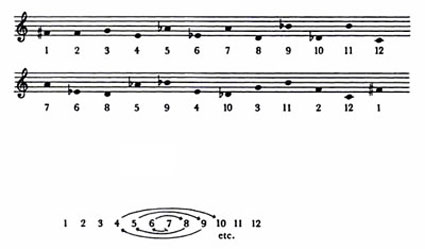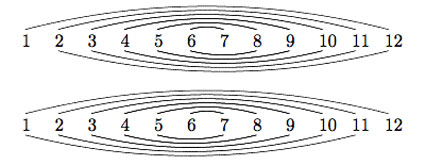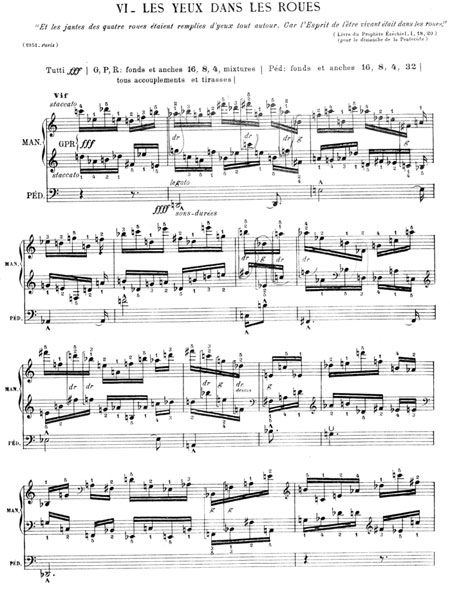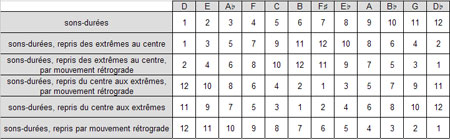To mark the end of 2009 and 6 years of blogging, two musical compositions with a mathematical touch to them. I wish you all a better 2010!

Remember from last time that we identified Olivier Messiaen as the ‘Monsieur Modulo’ playing the musical organ at the Bourbaki wedding. This was based on the fact that his “modes à transposition limitée” are really about epimorphisms between modulo rings Z/12Z→Z/3Z and Z/12Z→Z/4Z.

However, Messiaen had more serious mathematical tricks up his sleeve. In two of his compositions he did discover (or at least used) one of the smaller sporadic groups, the Mathieu group $M_{12}$ of order 95040 on which we have based a whole series of Mathieu games two and a half years ago.

Messiaen’s ‘Ile de fey 2’ composition for piano (part of Quatre études de rythme (“Four studies in rhythm”), piano (1949–50)) is based on two concurrent permutations. The first is shown below, with the underlying motive rotational permutation shown.This gives the permutation (1,7,10,2,6,4,5,9,11,12)(3,8). A second concurrent permutation is based on the permutation (1,6,9,2,7,3,5,4,8,10,11) and both of them generate the Mathieu group $M_{12}$. This can be seen by realizing the two permutations as the rotational permutationsand identifying them with the Mongean shuffles generating $M_{12}$. See for example, Dave Benson’s book “Music: A Mathematical Offering”, freely available online.

Clearly, Messiaen doesn’t use all of its 95040 permutations in his piece! Here’s how it sounds. The piece starts 2 minutes into the clip.

The second piece is “Les Yeux dans les Roues” (The Eyes in the Wheels), sixth piece from the “Livre d’Orgue” (1950/51).According to Hauptwerk, the piece consists of a melody/theme in the pedal, accompanied by two fast-paced homorhythmic lines in the manuals. The pedal presents a sons-durées theme which is repeated six times, in different permutations. Initially it is presented in its natural form. Afterwards, it is presented alternatively picking notes from each end of the original form. Similar transformations are applied each time until the sixth, which is the retrograde of the first. The entire twelve-tone analysis (pitch only, not rhythm) of the pedal is shown below:That is we get the following five permutations which again generate Mathieu 12 :

• a=(2,3,5,9,8,10,6,11,4,7,12)
• b=(1,2,4,8,9,7,11,3,6,12)(5,10)=e*a
• c=(1,12,11,9,5,4,6,2,10,7)(3,8)=e*d
• d=(1,11,10,8,4,5,3,7,2,9,6)
• e=(1,12)(2,11)(3,10)(4,9)(5,8)(6,7)

Here’s the piece performed on organ :

Considering the permutations $X=d.a^{-1}$ and $Y=(a.d^2.a.d^3)^{-1}$ one obtains canonical generators of $M_{12}$, that is, generators satisfying the defining equations of this sporadic group

$X^2=Y^3=(XY)^{11}=[X,Y]^6=(XYXYXY^{-1})^6=1$

I leave you to work out the corresponding dessin d’enfant tonight after a couple of glasses of champagne! It sure has a nice form. Once again, a better 2010!

### Similar Posts:

Published in stories